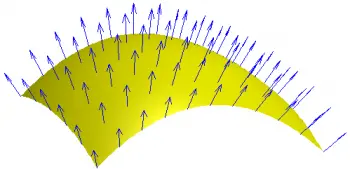# Magnetic Flux: Flux Density, Definition and FormulaMagnetic flux is defined as the physical quantity that describes the amount of magnetic field that passes through a given surface. It is a measure of how many magnetic field lines pass through a surface at a given angle.

To better understand this definition, imagine a region in space with a magnetic field, such as the field around a magnet. Now, let's place a flat surface in this region, which can be a real or imaginary surface, and which has a defined area.

## Formula

The magnetic flux through this surface is calculated by multiplying the value of the magnetic field at each point on the surface by the area of ​​that surface and then multiplying all by the cosine of the angle between the direction of the magnetic field and the normal vector to the surface. .

The formula that mathematically expresses this concept is as follows:

Φ = B * A * cos(θ)

Where:

• Φ is the magnetic flux in weber (Wb).

• B is the magnetic flux density in tesla (T).

• A is the surface area perpendicular to the magnetic field in square meters (m²).

• θ is the angle between the direction of the magnetic field and the normal vector to the surface.

Magnetic flux is measured in units called "Weber" (Wb) in the International System of Units (SI).

## Magnetic Flux Density

On the other hand, the magnetic flux density, which is represented by the letter "B", is a vector quantity that describes the intensity of the magnetic field at a specific point in space.

The magnetic flux density (B) at a point is related to the number of magnetic field lines passing through a surface perpendicular to the direction of the field.

Mathematically, the magnetic flux density is defined as the amount of magnetic flux (Φ) that passes through a unit area perpendicular to the magnetic field at that point:

B = Φ / A

Where:

• B is the magnetic flux density (magnetic induction) in tesla (T).

• Φ is the magnetic flux through a surface perpendicular to the field, measured in weber (Wb).

• A is the surface area perpendicular to the magnetic field in square meters (m²).

Its unit, the tesla, is equivalent to one weber per square meter (T = Wb/m²). Another commonly used unit to express magnetic flux density is the gauss, where 1 T = 10,000 gauss (1 mT = 1 gauss).

## Instruments for Measuring Magnetic Flux

There are various instruments and devices used to measure magnetic flux in various applications. Some of the most common instruments are:

1. Gaussmeter Gauges: measure in units of Gauss (G). They are widely used to measure magnetic fields in industrial applications, such as inspection of magnets and magnetic materials.

2. Teslameter meters: measure in units of Tesla (T). They are more accurate than gaussmeters and are used in more specialized applications, such as in research laboratories and scientific studies.

3. Helmholtz Coil: This is a configuration of two identical coils placed at a specific distance from each other. It is used to generate known, uniform magnetic fields in a defined area, allowing other measuring devices to be calibrated and verified.

4. Magnetic flow sound level meters: These are specialized instruments that use vibration effects or sounds produced by ferromagnetic objects in a magnetic field. These sounds are correlated with the flow density and allow indirect measurements to be made.

5. Inductors: These devices are used to measure the inductance in coils, and from this measurement, it is possible to obtain information about the magnetic flux present.

6. Hall Sensors: Hall sensors are semiconductor devices that can directly measure flux density. They are used in applications such as the detection of magnetic fields in motors, electronic devices and navigation systems.

7. Magnetometers: are instruments that measure the intensity and direction of the magnetic field at a specific point.

## Applications That Use Magnetic Flux

Knowledge of magnetic flux is essential in various applications and fields of science and technology. Some of the main applications where it is important to know the magnetic flux are the following:

1. Electrical industry and power generation: In the electrical industry it is used in generators and transformers for the production, transmission and distribution of electrical energy.

2. Electronics and semiconductor technology: Some devices such as magnetic sensors, microchips, hard drives, and other components use magnetic fields to function.

3. Medicine: In medicine, magnetic resonance imaging (MRI) is used to obtain detailed information about tissues and organs in the human body. Magnetic resonance is based on the manipulation and detection of the magnetic flux generated by hydrogen atoms in the body.

4. Geophysics and navigation: In geophysics, the study of the Earth's magnetic field helps to understand the internal structure of the planet. Also, in navigation, compasses use magnetic flux to determine direction.

5. Control and stabilization of systems: In some applications, such as the stabilization of control systems, it is important to know and compensate for the effects of magnetic fields to avoid interference or undesired effects.

6. Metal and materials industry: Knowledge of magnetic flux is useful in quality testing, inspection of ferromagnetic materials, and manufacturing process control.

7. Transportation and magnetic levitation: In transportation applications, such as magnetic levitation (Maglev) trains, this physical property is used for propulsion and control of the vehicle without physical contact with the tracks through electromagnets.

Author:

Published: July 26, 2023
Last review: July 26, 2023